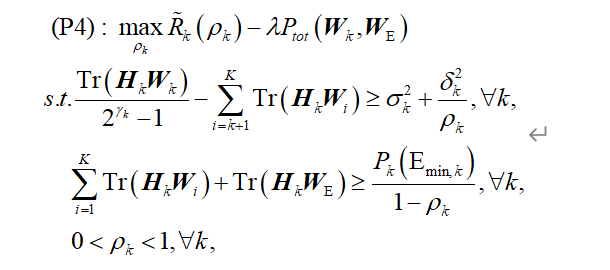# What is causing the final Status: Inaccurate/Solved?%%%%%%%%%% CVX2 Optimize Power Splitting Factor
cvx_begin sdp quiet
% cvx_precision low
variable rho(K)
variable afa(K)
variable bta(K)
N2 = 0;
for k = 1:K
chnl00 = 0;
for i =1:K
chnl00 = chnl00 + real(trace(H_k(:,:,k)W(:,:,i)));
end
afa(k) >=inv_pos(rho(k));
N2 = N2 +log(chnl00 +sigma + derta
afa(k))/log(2);%%%%%引入松弛变量 %%inv_pos(rho(k)) ;1/(rho(k)/derta);log(chnl00 +sigma + derta*1/(rho(k)))/log(2)
end
D2_pre = 0;
for k = 1:K
chnl11 = 0;
for i = k+1:K
chnl11 = chnl11 + real(trace(H_k(:,:,k)W(:,:,i)));
end
D2_pre = D2_pre +log(chnl11+sigma+derta
(1/rho_pre(k)))/log(2);%%%%一阶泰勒展开第一项
end

r_22 = 0;
D2 = 0;
for k = 1:K
r_21 = 0;
for i = k+1:K
r_21=r_21 + real(trace(H_k(:,:,k)W(:,:,i)));
end
r_22 = r_22 +((rho(k)-rho_pre(k))derta)/log(2)((rho_pre(k)^2)
(r_21+sigma)+rho_pre(k)*derta);
end
D2 = D2_pre - r_22;
result1 = (N2 - D2)/P_total;
maximize result1
subject to
%%%%%%%%%%%%%R_min Qos Constraint

for k =1:K
r_C1 = 0;
for i =k+1:K
r_C1 = r_C1 + real(trace(H_k(:,:,k)*W(:,:,i)));
end
real(trace(H_k(:,:,k)*W(:,:,k)))/(2^R_min - 1) -r_C1 >= sigma + derta *afa(K);
end
%%%%%%%%%%%%%Energy Qos Constraint

for k = 1:K
r_C2 = 0;
for i =1:K
r_C2 = r_C2 + real(trace(H_k(:,:,k)*W(:,:,i))) ;
end
bta(k)>=inv_pos(1-rho(k));
r_C2 + real(trace(W_E)) >=real(E_NL)*bta(k);
end
for k =1:K
0<=rho(k)<=1;
end
cvx_end

CVX Warning:
Models involving “log” or other functions in the log, exp, and entropy
family are solved using an experimental successive approximation method.
This method is slower and less reliable than the method CVX employs for
other models. Please see the section of the user’s guide entitled
The successive approximation method
for more details about the approach, and for instructions on how to
suppress this warning message in the future.

## Cones | Errors | Mov/Act | Centering Exp cone Poly cone | Status --------±--------------------------------±-------- 3/ 3 | 6.632e-01 3.337e-02 0.000e+00 | Inaccurate/Solved 3/ 3 | 1.735e-02 2.181e-05 0.000e+00 | Inaccurate/Solved 2/ 3 | 1.077e-03 8.362e-08 0.000e+00 | Inaccurate/Solved 0/ 0 | 0.000e+00 0.000e+00 0.000e+00 | Inaccurate/Solved 0/ 0 | 0.000e+00 0.000e+00 0.000e+00 | Inaccurate/Solved 0/ 0 | 0.000e+00 0.000e+00 0.000e+00 | Inaccurate/Solved

Status: Inaccurate/Solved
Optimal value (cvx_optval): +25.1737

204 cvx_begin sdp quiet

I don’t see any `log` in P4. Why is there `log in your program? Are some of the things in P4 actually log of some function of the optimization variables?

Bad numerical scaling is a likely contributor to why you got Inaccurate/solved… You should check the input data.

If you have Mosek, use that as solver, otherwise, follow the advice at CVXQUAD: How to use CVXQUAD's Pade Approximant instead of CVX's unreliable Successive Approximation for GP mode, log, exp, entr, rel_entr, kl_div, log_det, det_rootn, exponential cone. CVXQUAD's Quantum (Matrix) Entropy & Matrix Log related functions . That may help.

And don’t use `quiet` mode until everything is working well.